## Shewhart Charts

While working for Western Electric in the 1920s, Dr. Shewhart developed a general, practical approach to statistical monitoring of manufacturing processes.  He advised managers on implementing these within Western Electric and later published his work in Shewhart (Montgomery, 1931).  All Shewhart control charts share several characteristics in common.  First, the horizontal axis represents time or lot sequence, but they all have different vertical axes, depending upon the chart time.

Next, all Shewhart control charts have a center line that is drawn parallel to the time axis. This typically represents the mean of the process, but the value of the process mean can vary depending upon which data are first used to design the chart. In some cases it will be the mean of the data plotted, in others it could be the mean calculated from a much larger number of measurements on the historical operation of the process.

Lastly, all Shewhart control charts have lines drawn to represent either the upper or lower control limits. In most cases both control lines are present, in others where the data have a natural bound, such as zero, only one of these control limits might be drawn.

Shewhart control charts are also broadly classified into two groups:  variable and attribute data charts. Variable control charts are used when the quality of interest is a continuous variable, such as the diameter of a valve. If w is a continuous measure of a quality of interest, with mean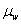and within-sample standard deviation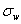, then the center line is atand the upper and lower controls limits are at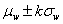. Typically k = 3 and the charts are called 3-sigma control charts.

Attribute control charts are used when qualities, not quantities are measured. For example, items may be characterized as conforming or nonconforming to a specification. Items may also be characterized as defective or nondefective. Examples of attributes include the number of failures in a manufacturing run or the number of defects on a computer chip wafer.

When the number of defective or nondefective items are plotted then a PChart or an NpChart are generally used to describe the non-comformity data. The NpChart is used to plot the number of defects when all of the sample sizes are equal, and the PChart is used when the sample sizes are unequal.

If a single item can have multiple defects then a CChart or UChart is used, depending upon whether the area inspected for defect is consistent or varying. An example of multiple defects per item would be the count of the number of scratches on mirrors. If all samples have an equal opportunity for defects use CChart, otherwise use UChart. So to monitor the number of scratches on mirrors use CChart when all mirrors being made are the same size and use UChart for mirrors being made that are sized differently.

In IMSL C# Numerical Library the ShewhartControlChart class is the base of a number of classes; it is not usually used by itself. Most of the charts in this chapter extend ShewhartControlChart.

The following diagram can be used to determine the appropriate control chart to be used in a given situation.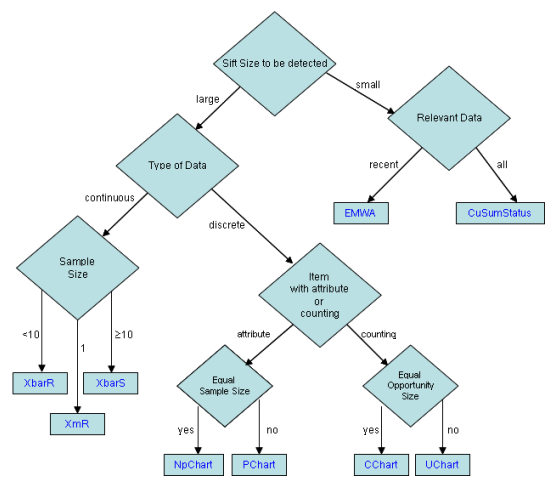Variable Control Charts:

XbarR estimatesandusing the ranges of the samples. It is best used when the sample size of a continuous variable is between 2 and 10.

RChart plots the sample ranges. It is typically used in conjunction with XbarR.

XbarS estimatesandusing the means and standard deviations of the samples. It is best used when the sample size of a continuous variable is at least 10.

SChart plots the sample standard deviations. It is typically used in conjunction with XbarS.

XmR is a moving range chart. It is used when the sample size of a continuous variable is one.

EWMA (Exponentially Weighted Moving-Average) plots weighted moving average values. It is used when the sample size of a continuous variable is one.

Attribute Control Charts:

NpChart plots the number of defects. It is used when defects are not rare.

PChart plots the rate of defects. It is used when defects are not rare.

CChart plots the defect count. It is used when defects are rare.

UChart plots the rate of defects. It is used when defects are rare.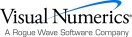Visual Numerics - Developers of IMSL and PV-WAVEhttp://www.vni.com/PHONE: 713.784.3131FAX:713.781.9260